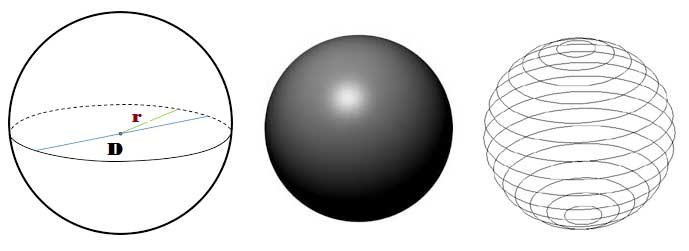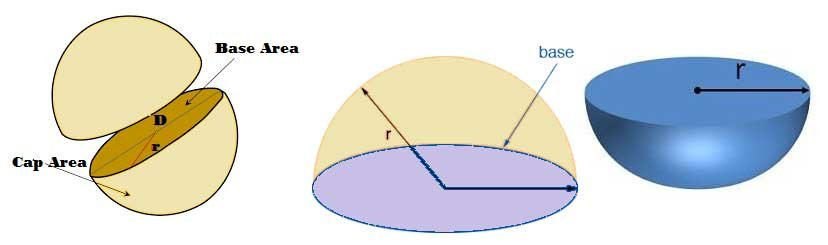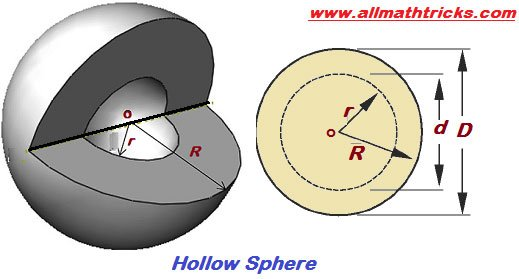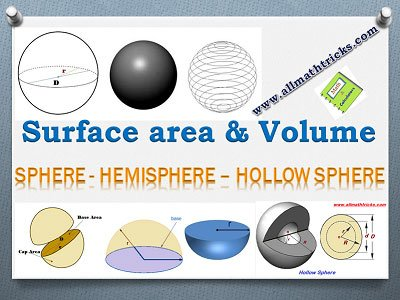# Surface Area and Volume of Sphere, Hemisphere, Hollow Sphere Formulas, Examples

In this article provided formulas of Surface Area and Volume of a Sphere and a Hemisphere with examples. Volume and surface area of a three dimensional (3D) solid geometrical shapes.

Contents

## Sphere and Hemisphere Formulas with Examples

### Sphere

Sphere is a one of the three dimensional solid figure, Which made up of all points in the space, Which lie at a constant distance called the radius, from a fixed point called the center of a sphere. ( i.e The set of all points in the three space equidistant from a given points form sphere)#### Surface Area of a Sphere

Surface area of the sphere will be covered completely fill the region of four circles, all of the same radius as of the sphere. So

Surface area of a Sphere with radius ( r )  = 4 x ( π r2 ) = 4 π r2

#### Volume of a Sphere

Volume of a sphere is equal to 4 π/3 times the cube of its radius.

Volume of a sphere =### Hemisphere

The solid sphere is divided into two equal parts and its each half part is called a hemisphere.#### Surface Area of a Hemisphere

Surface area of the hemisphere having two faces. There is curved face (Cap Area) and flat face ( base area).

Surface area of a Sphere with radius ( r )  = 4 π r2

Then cap area of hemisphere is half surface area of the sphere

i.e Cap Area or Curved surface area of the hemisphere = 1/2 ( 4 π r2 ) = 2 π r2

Flat surface area or base area of the hemisphere = Area of the circle with same radius = π r2

Total Surface Area of the Hemisphere = 3 π r2

#### Volume of a Hemisphere

Hemisphere is half of a sphere

Volume of a hemisphere  = ( 1/2 ) ( 4 /3 π r2 ) =#### Surface Area and Volume of Hemisphere shell

Take external radius is ‘R’ and inner radius is ‘r’ of hemisphere

then

Curved surface area of hemisphere shell = 2 π  ( R2 + r2 ) ( Considered inside and outside area of hemisphere)

Volume of Hemisphere shell =### Volume of Hollow SphereHere Hollow sphere inner radius – r & outer radius – Rr

Volume of allow sphere =Solid Sphere

Solid Sphere is the region in space bound by a sphere.

### Examples on surface area and volume of sphere and hemisphere

Example -1 : Find the surface area and volume of sphere having the radius 7 mm

Solution: Here radius of sphere  = r = 7 mm

Now Surface area of a Sphere = 4 π r2

= 4 x (22/7) x 7 x 7 = 616 mm2

Volume of a sphere == (4312/3 ) mm3

Example-2 : A shot-put is a metallic sphere of radius 2.1 cm and density of the metal used for same is 7.8 gm/cm3. Find the weight of the shot-put

Solution: The shot-put is a solid sphere with the radius 2.1 cm

Volume of shot-put == (4/3) x (22/7) x 2.1 x 2.1 x 2.1 = 38.8o8 cm3

Density of metal used for making shot-put is 7.8 gm/cm3

Weight of the shot-put = 38.8o8 x 7.8 ( gm x cm3 /cm3 ) = 302.7 gm

Example-3 : The volume of a solid hemisphere is 18π mm3. Find the total surface of the same.

Solution: Take the radius of sphere = r, then

Volume of a sphere =⇒= 18π

⇒ r = 3

Total Surface area of hemisphere = 3 π r2 = 27 π

Example-4: The three metallic spheres have radii 3cm, 4cm & 5cm respectively, are melted to form a single solid sphere. Find the radius of the resulting sphere.

Solution: Take the radius of final sphere is R

Volume of final sphere = volume of individual spheres

⇒ (4/3) x π x R3 = (4/3) x π x 33 + (4/3) x π x 43 + (4/3) x π x 53

R3 = ( 33 + 43 + 53 ) = 63

R = 6

Example-5 : A hemispherical bowl has a radius of 4.2 cm. What would be the volume of mercury it would contain?

Solution: Here radius of hemispherical bowl = r = 4.2 cm

Volume of a hemisphere  == (2/3) x (22/7) x 4.2 x 4.2 x 4.2 = 155.232 cm3

Note: Here asked only volume of mercury. In case of ask mass of the mercury then multiplying with density with volume)

Example-6: A metallic sphere have diameter of 6 cm. The metallic sphere is melted and manufacturing into a wire of uniform cross section. Find the radius of wire if the length of the wire is 36 m.

Solution: Here radius of sphere = r = 3 cm &  Length of wire = 36 m = 3600 cm

Volume of Sphere = Volume of wire

⇒ (4/3) π r= π r2 h

⇒ ( 4/3)  x ( 22/7) x 3 x 3 x 3   = (22/7) x r2 x 3600

⇒ r = 1/10 cm = 1 mm

Example-7: Volumes of two spheres are in the ratio 125:64. Then find the ratio of their surface areas.

Solution: Take radius of spheres are r1 and r2

Ratio of volume of Spheres = 125 : 64= 125 : 64

⇒ r: r2 = 5 : 4

Ratio of surface area of spheres= r1 2: r2 2 = 52 : 4 = 25 : 16

Example-8: A hemisphere tank is fabricated with an iron sheet of 1 cm thick. If the inner radius is 10 cm , then find the weight of the iron used to make the tank. (To be take Density of iron = 7 gm/cm3)

Solution: Hemisphere inner radius = 9 cm,then outer radius = 10 cm

Volume of shell of hemisphere == ( 2/3 )x (22/7) x ( 103 – 93) = ( 2/3 )x (22/7) x (1000 – 729) = (11924/21) cm3

Weight of the iron required = (11924/21) x 7 = (11924 /3) = 3974.66 gmRelated Articles

Area, Circumference, Sector, Chord, Arc of Circle

Formulas of Rectangle, Square, Trapezium, parallelogram, Rhombus, kite

Lines, line segment and ray

Interior angles, Exterior Angles, Alternate Interior & Alternate Exterior Angles

## 1 thought on “Surface Area and Volume of Sphere, Hemisphere, Hollow Sphere Formulas, Examples”#### filmi full izle

(January 16, 2021 - 11:45 pm)

Hiya, I am really glad I have found this information. Nowadays bloggers publish only about gossip and net stuff and this is actually frustrating. Pattie Vaclav Gleda